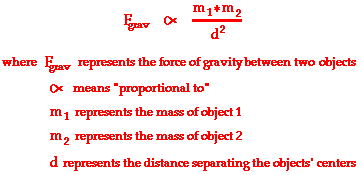# Gravitational ForceNewton's Law of Universal Gravitation describes how ALL objects attract each other's mass with a force of gravitational attraction.

The force of gravitational attraction was found experimentally to be directly proportional to the masses of each of the objects and inversely proportional to the square of the distance that separates their centers.To make this into an equation we need to add a 'constant of proportionality'. This is 'G' - the gravitational constant. The precise value of G was determined experimentally by Henry Cavendish in 1798, almost a century after Newton's death.  It has a value of 6.673 x 10-11 N m2 kg-2, and you will find it on your data sheet in examinations. It is also known as the universal gravitational constantNewton's constant, and colloquially as 'Big G'.

In the AQA A level syllabus the distance between the masses is denoted by 'r'.Mass m1 is pulling mass m2, and mass m2 is pulling mass m1... newton's third law!